# 如何用Python读取Excel中的图片？然后写入图片？

• Python读取Excel图片
• Python写入Excel图片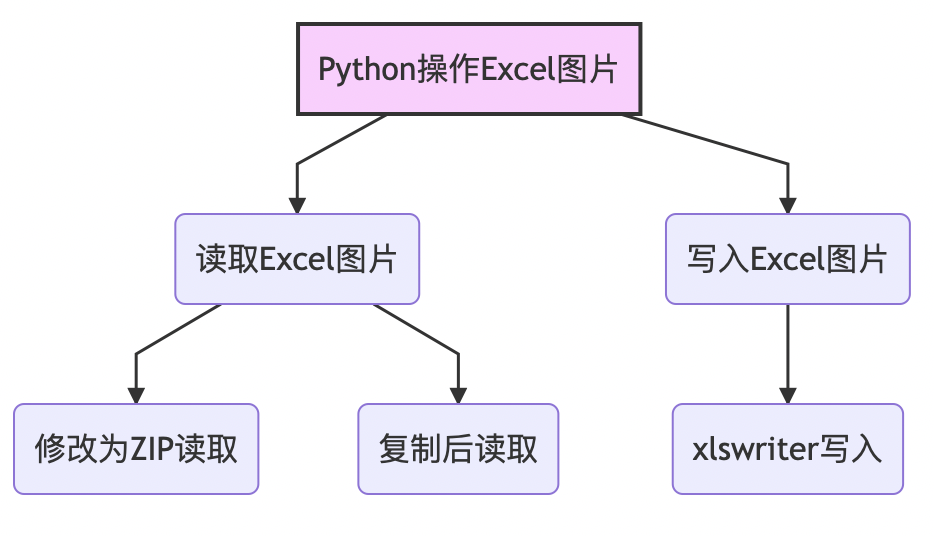• PIL
• win32
• os
• zipfile
• numpy
• xlsxwriter

一、准备

pip install pillow   #这是对模块PTL的安装
pip install pypiwin32    #这是对win32的安装
pip install os
pip install zipfile
pip install numpy
pip install xlsxwriter

http://pypi.douban.com/simple/ 豆瓣
http://mirrors.aliyun.com/pypi/simple/ 阿里
http://pypi.hustunique.com/simple/ 华中理工大学
http://pypi.sdutlinux.org/simple/ 山东理工大学
http://pypi.mirrors.ustc.edu.cn/simple/ 中国科学技术大学
https://pypi.tuna.tsinghua.edu.cn/simple 清华

pip install 模块名 -i 网址

pip install pillow -i https://pypi.tuna.tsinghua.edu.cn/simple

二、Python读取Excel图片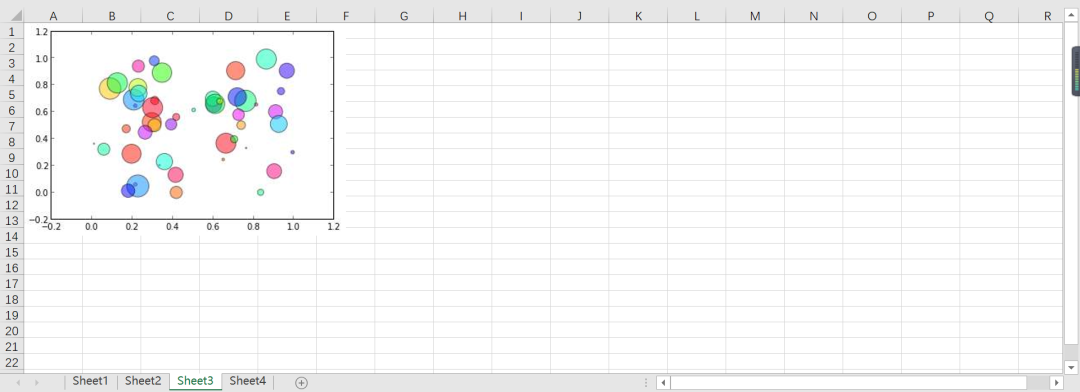2.1  方法一

import os
import zipfile
import os
from PIL import Image
import numpy as np
path = r'D:'
count = 1
for file in os.listdir(path):
new_file = file.replace(".xlsx",".zip")
os.rename(os.path.join(path,file),os.path.join(path,new_file))
count+=1
print('总共有'+str(count)+'个文件夹')
number = 0
craterDir = "D:/"  # 存放zip文件的文件夹路径
saveDir = "D:/"  # 存放图片的路径
list_dir = os.listdir(craterDir)
for i in range(len(list_dir)):
if 'zip' not in list_dir[i]:
list_dir[i] = ''
while '' in list_dir:
list_dir.remove('')
for zip_name in list_dir:
print(zip_name)
azip = zipfile.ZipFile(craterDir + zip_name)
namelist = (azip.namelist())

for idx in range(0,len(namelist)):
if namelist[idx][:9] == 'xl/media/':#图片是在这个路径下
img_name = saveDir + str(number)+'.jpg'
f = azip.open(namelist[idx])
img = Image.open(f)
img = img.convert("RGB")
img.save(img_name,"JPEG")
number += 1
azip.close()  #关闭文件，必须有，释放内存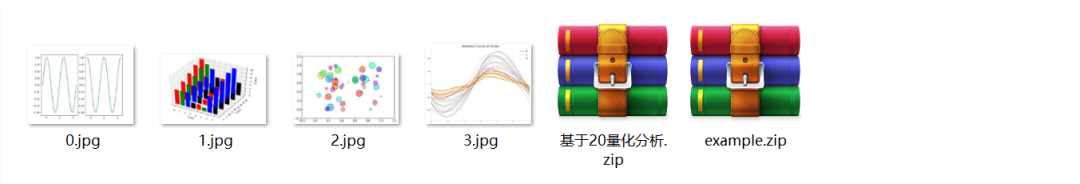import os
import zipfile
from PIL import Image
import numpy as np


path = r'D:'   #excel文件位置
count = 1
for file in os.listdir(path):
new_file = file.replace(".xlsx",".zip")
os.rename(os.path.join(path,file),os.path.join(path,new_file))
count+=1


number = 0
craterDir = "D:/"  # 存放zip文件的文件夹路径
saveDir = "D:/"  # 存放图片的路径
list_dir = os.listdir(craterDir) # 获取所有的文件名

for i in range(len(list_dir)):
if 'zip' not in list_dir[i]:
list_dir[i] = ''
while '' in list_dir:
list_dir.remove('')


for zip_name in list_dir:
print(zip_name)
# 默认模式r,读
azip = zipfile.ZipFile(craterDir + zip_name)
# 返回所有文件夹和文件
namelist = (azip.namelist())

for idx in range(0,len(namelist)):
if namelist[idx][:9] == 'xl/media/':#图片是在这个路径下
img_name = saveDir + str(number)+'.jpg'
f = azip.open(namelist[idx])
img = Image.open(f)
img = img.convert("RGB")
img.save(img_name,"JPEG")
number += 1
azip.close()  #关闭文件，必须有，释放内存


azip.open()是zipfile模块里的打开命令。相对应的，就有azip.close()这个命令，这个命令再整个程序运行完后必须运训，因为不仅可以清理所占空间，而且如果你需要还原xlsx文件格式的话，你就必须得关闭，不然会报错。

Image.open()是模块Pillow模块的读取图片函数，也算是我们本章最重要的函数之一了，与其搭配的是Image.save()函数，是用来存储的。中间插着一段代码img.convert("RGB")，这个一般都是要用到的，我们存储的图片大多数是有色的，也就是RGB图像，如果是黑白的话就要调一下参数。有兴趣的读者可以自行查阅文献。

2.2  方法二

from PIL import ImageGrab
import win32com.client as win32

excel = win32.gencache.EnsureDispatch('Excel.Application')
workbook = excel.Workbooks.Open(r'D:\example.xlsx')

num = 1
for sheet in workbook.Worksheets:
for i, shape in enumerate(sheet.Shapes):
if shape.Name.startswith('Picture'):
shape.Copy()
image = ImageGrab.grabclipboard()
image.convert('RGB').save(r'D:\{}.jpg'.format(num), 'jpeg')
num+=1
excel.Quit()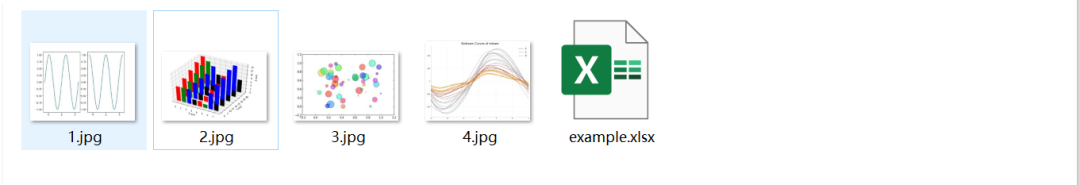from PIL import ImageGrab
import win32com.client as win32
excel = win32.gencache.EnsureDispatch('Excel.Application')
workbook = excel.Workbooks.Open(r'D:\example.xlsx')


num = 1
for sheet in workbook.Worksheets:
for i, shape in enumerate(sheet.Shapes):
if shape.Name.startswith('Picture'):
shape.Copy()
image = ImageGrab.grabclipboard()
image.convert('RGB').save(r'D:\{}.jpg'.format(num), 'jpeg')
num+=1
excel.Quit()


shape.Name.startswith('Picture')是判断获到的shape里面名字信息中的开头是否符合'pitcure'，如果是，则返回True。

shape.Copy()函数是对一个一个字典的浅复制（拷贝）。简单来说就是只在程序中的复制。

ImageGrab.grabclipboard()函数是方法二的精髓，这个函数是抓取当前剪贴板的快照，返回一个模式为“RGB”的图像或者文件名称的列表。如果剪贴板不包括图像数据，这个函数返回空。读者可以使用函数isinstance()来检查该函数返回的是一个有效图像对象或者其他数据。

image.convert('RGB').save(r'D:\{}.jpg'.format(num), 'jpeg')这段语句，是将得到的image以jpg的格式存储。这里的jpeg其实就是jpg，只不过模块里以jpeg代替jpg。

二、Python写入Excel图片

worksheet.insert_image(row,col,image[,options] ：在工作表单元格中插入一张图片

row(int) - 单元格所在的行（从0开始）

col(int) - 单元格所在的列（从0开始）

image(string) - 图片文件名（含路径）

options(dict) - 可选的图片位置，缩放，url参数

{
'x_offset': 0,    #以像素为单位，可以大于每个单元格的宽度和高度
'y_offset': 0,
'x_scale': 1,
'y_scale': 1,
'url': None,
'tip': None,
'image_data': None,
'positioning': None,
}

x_scale和y_scale参数可以用于水平及垂直的缩放图片。

url参数可以为图片添加超链接/url， tip 参数为含有超链接的图片提供可选的鼠标悬停时的提示信息

image_data参数用于在io.BytesIO中添加内存中的字节流，一般不用

positioning参数可以用来控制图片对象的位置

import pandas as pd
import matplotlib.pyplot as plt
import matplotlib.style as psl
import xlsxwriter
fig = plt.figure()
df = pd.read_excel(r'D:\数据.xlsx')
title = ['日期','收盘价']
df = df[title]
plt.plot(df['日期'],df['收盘价'])
plt.gcf().autofmt_xdate()#自动调整角度
plt.savefig(r'D:\数据折线图.jpg')


Python画出的图如下：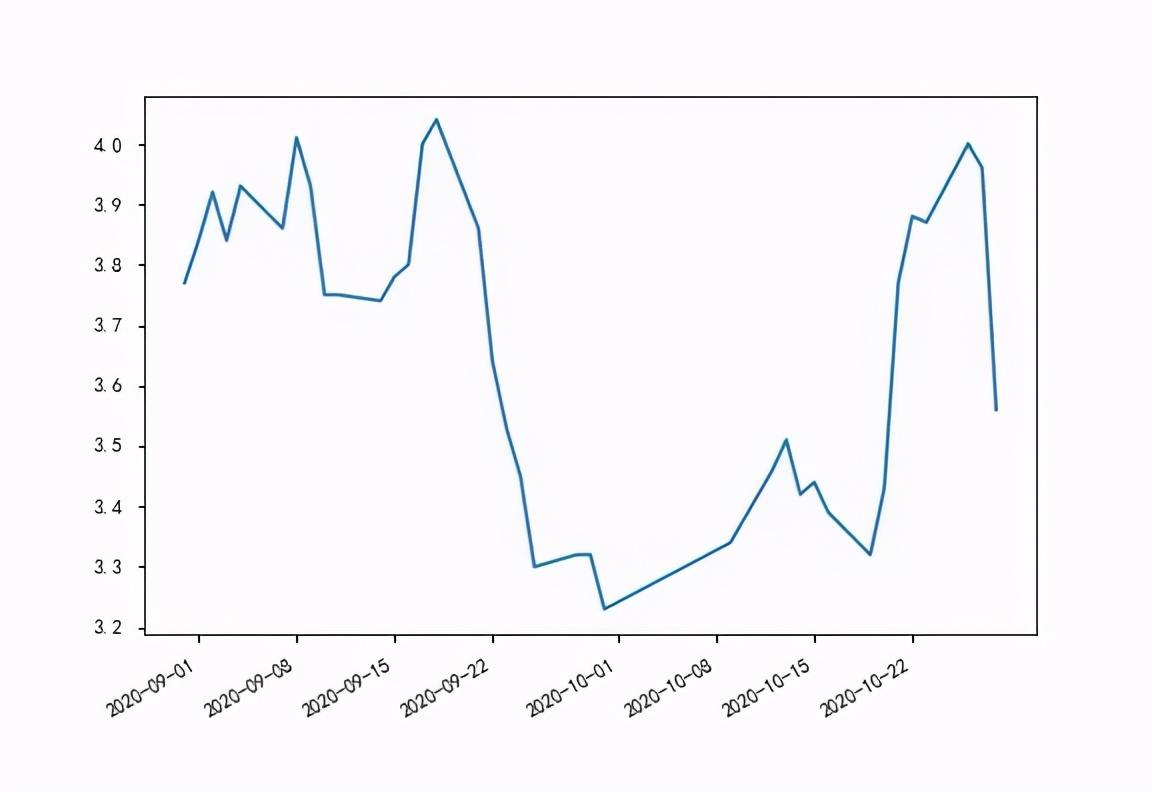workbook = xlsxwriter.Workbook(r'D:\数据images.xlsx')
worksheet = workbook.add_worksheet()


worksheet.write('A2', '插入第一张图片：')
worksheet.insert_image('B2', r'D:\数据折线图.jpg')

worksheet.write('A12', '插入第二张即位偏移图片：')
worksheet.insert_image('B12', r'D:\数据折线图.jpg', {'x_offset': 15, 'y_offset': 10})

# 插入一张缩放了的图片。
worksheet.write('A23', '插入第三张缩放了的图片：')
worksheet.insert_image('B23', r'D:\数据折线图.jpg', {'x_scale': 0.5, 'y_scale': 0.5})

workbook.close()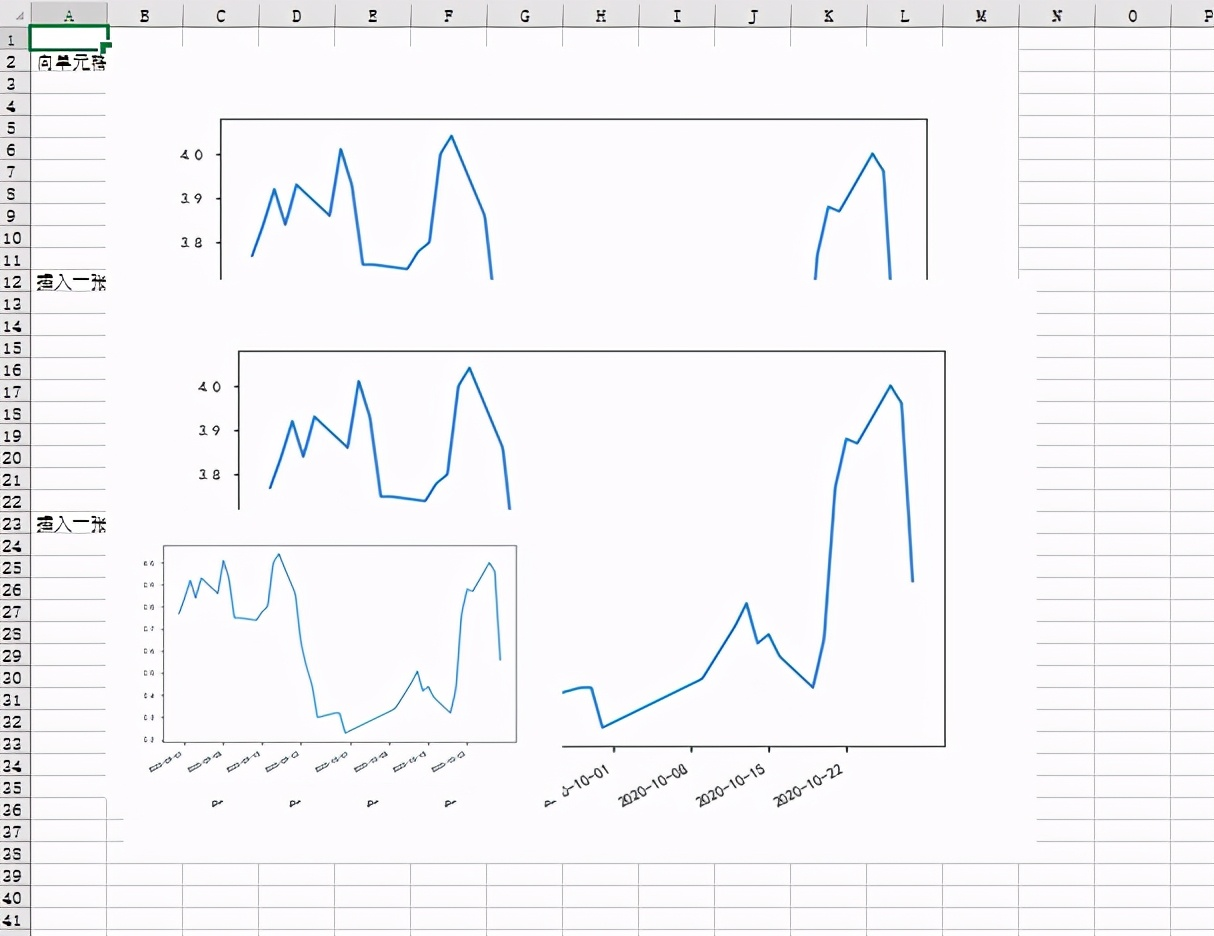08-051万+
06-19275
06-191595
03-30960
05-147295
06-2918万+
07-261万+
01-082927
11-191181
11-183406
02-222578
©️2020 CSDN 皮肤主题: 精致技术 设计师:CSDN官方博客